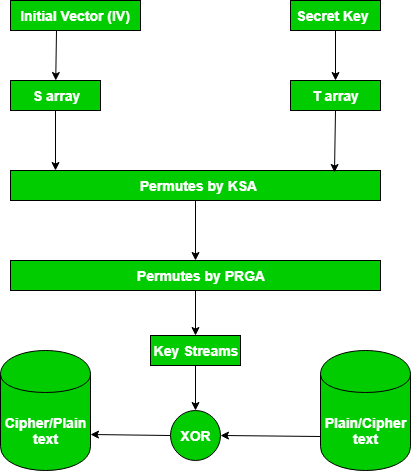# RC4 Encryption Algorithm

RC4 is a stream cipher and variable length key algorithm. This algorithm encrypts one byte at a time (or larger units on a time).
A key input is pseudorandom bit generator that produces a stream 8-bit number that is unpredictable without knowledge of input key, The output of the generator is called key-stream, is combined one byte at a time with the plaintext stream cipher using X-OR operation.
Example:

```RC4 Encryption
10011000 ? 01010000 = 11001000

RC4 Decryption
11001000 ? 01010000 = 10011000
```

Key-Generation Algorithm –
A variable-length key from 1 to 256 byte is used to initialize a 256-byte state vector S, with elements S to S. For encryption and decryption, a byte k is generated from S by selecting one of the 255 entries in a systematic fashion, then the entries in S are permuted again.

1. Key-Scheduling Algorithm:
Initialization: The entries of S are set equal to the values from 0 to 255 in ascending order, a temporary vector T, is created.
If the length of the key k is 256 bytes, then k is assigned to T. Otherwise, for a key with length(k-len) bytes, the first k-len elements of T as copied from K and then K is repeated as many times as necessary to fill T. The idea is illustrated as follow:

 `for` `    ``i = ``0` `to ``255` `do` `S[i] = i; ` `T[i] = K[i mod k - len]; `

we use T to produce the initial permutation of S. Starting with S to S, and for each S[i] algorithm swap it with another byte in S according to a scheme dictated by T[i], but S will still contain values from 0 to 255 :

 `j = ``0``; ` `for` `    ``i = ``0` `to ``255` `do` `    ``{ ` `        ``j = (j + S[i] + T[i])mod ``256``; ` `        ``Swap(S[i], S[j]); ` `    ``} `

2. Pseudo random generation algorithm (Stream Generation):
Once the vector S is initialized, the input key will not be used. In this step, for each S[i] algorithm swap it with another byte in S according to a scheme dictated by the current configuration of S. After reaching S the process continues, starting from S again

 `i, j = ``0``; ` `while` `(``true``) ` `    ``i = (i + ``1``)mod ``256``; ` `j = (j + S[i])mod ``256``; ` `Swap(S[i], S[j]); ` `t = (S[i] + S[j])mod ``256``; ` `k = S[t]; `

3. Encrypt using X-Or():News:
In September 2015, Microsoft announced the end of using RC4 in Microsoft edge and internet explorer 11.

This video gives a clear example of RC4 algorithm

Attention reader! Don’t stop learning now. Get hold of all the important CS Theory concepts for SDE interviews with the CS Theory Course at a student-friendly price and become industry ready.

My Personal Notes arrow_drop_upCheck out this Author's contributed articles.

If you like GeeksforGeeks and would like to contribute, you can also write an article using contribute.geeksforgeeks.org or mail your article to contribute@geeksforgeeks.org. See your article appearing on the GeeksforGeeks main page and help other Geeks.

Please Improve this article if you find anything incorrect by clicking on the "Improve Article" button below.

Improved By : Akanksha_Rai

Article Tags :
Practice Tags :

1

Please write to us at contribute@geeksforgeeks.org to report any issue with the above content.